# August 2021 - Los Angeles, CA

ACADEMIC CREDENTIALS EVALUATION INSTITUTE, INC. (ACEI)
Founded in 1994, ACEI is a full-service company providing complete and integrated services in-house in the areas of international education research, credential evaluation, and translation. And, through our Global Consulting Group®, we offer our expertise in the following specialties: Media and Branding, Global Pathways, and Diversity, Equity and Inclusion (DEI) to interested institutions and organizations around the globe.

 table div table+table+table div table{width:100%;padding:0}table div table+table+table div table img{width:96.23%;padding:0;float:none}table div table+table+table div table td{width:100%;padding:0 1.88% 18px}/* styles */table.module-3{width:81.7%;padding:0}table div table+table+table+table div table{width:81.7%;float:none;margin-left:auto;margin-right:auto;padding:0}table div table+table+table+table div table a{border:0 none;text-decoration:none}table div table+table+table+table div table img{width:100%!important;border:0 none;text-decoration:none}table div table+table+table+table div table td{width:100%;padding:0}/* styles */

# ACEI Global Consulting Group

The Global Consulting Group is a division of ACEI that provides international education expertise with focus on Diversity, Equity, Inclusion Consulting, Global Pathway Consulting (Program/Curriculum Review for Partnerships between overseas institutions), and Media and Brand Consulting. Click here to view video presentation.

 table div table+table+table+table+table+table div table{width:100%;padding:0}table div table+table+table+table+table+table div table img{width:96.23%;padding:0;float:none}table div table+table+table+table+table+table div table td{width:100%;padding:0 1.88% 18px}/* styles */# The Report Partners

 table div table+table+table+table+table+table+table+table div table{width:100%;padding:0}table div table+table+table+table+table+table+table+table div table table{padding:0;float:left!important;width:28.679%!important}table div table+table+table+table+table+table+table+table div table table+table+table td,table div table+table+table+table+table+table+table+table div table td{padding-left:0;padding-right:0}table div table+table+table+table+table+table+table+table div table table td,table div table+table+table+table+table+table+table+table div table table+table td{padding-left:0;padding-right:20px}table div table+table+table+table+table+table+table+table div table table+table{float:left!important;width:41.698%!important}table div table+table+table+table+table+table+table+table div table table+table+table{float:left!important;width:29.623000000000005%!important}/* styles */
 table div table+table+table+table+table+table+table+table+table div table{width:100%;padding:0}table div table+table+table+table+table+table+table+table+table div table img{width:96.23%;padding:0;float:none}table div table+table+table+table+table+table+table+table+table div table td{width:100%;padding:0 1.88% 18px}/* styles */# ACEI News

 /* styles */ Missed an ACEI webinar? You can access recordings of our webinars via this link
 table div table+table+table+table+table+table+table+table+table+table+table+table div table{width:100%;padding:0}table div table+table+table+table+table+table+table+table+table+table+table+table div table img{width:96.23%;padding:0;float:none}table div table+table+table+table+table+table+table+table+table+table+table+table div table td{width:100%;padding:0 1.88% 18px}/* styles */# ACEI-Global.Blog

## 3 Tips for Stakeholder Engagement to Make the Most of Fall In-Service/* styles */ Whether you are heading into or out of your Fall In-Service to get the 2021/22 academic year off to a great start, the importance of getting the most buy-in and engagement from all of your program’s stakeholders I something that’s probably top of mind. To read more, click here.
 table div table+table+table+table+table+table+table+table+table+table+table+table+table+table+table+table+table div table{width:100%;padding:0}table div table+table+table+table+table+table+table+table+table+table+table+table+table+table+table+table+table div table img{width:96.23%;padding:0;float:none}table div table+table+table+table+table+table+table+table+table+table+table+table+table+table+table+table+table div table td{width:100%;padding:0 1.88% 18px}/* styles */# Education News From Around the GlobePhoto credit: Jim Huylebroek/The New York Times/Redux

# As Taliban Advances, the Future of Education is Uncertain

Never in the history of Afghanistan’s conservative and remote corners have so many girls made it into higher education as in the past few years of relative peace. Now, amid swift and deadly advances by armed Taliban insurgents, these gains – and their lives – are under threat. To read more, click here.

 table div table+table+table+table+table+table+table+table+table+table+table+table+table+table+table+table+table+table+table+table+table div table{width:100%;padding:0}table div table+table+table+table+table+table+table+table+table+table+table+table+table+table+table+table+table+table+table+table+table div table img{width:96.23%;padding:0;float:none}table div table+table+table+table+table+table+table+table+table+table+table+table+table+table+table+table+table+table+table+table+table div table td{width:100%;padding:0 1.88% 18px}/* styles */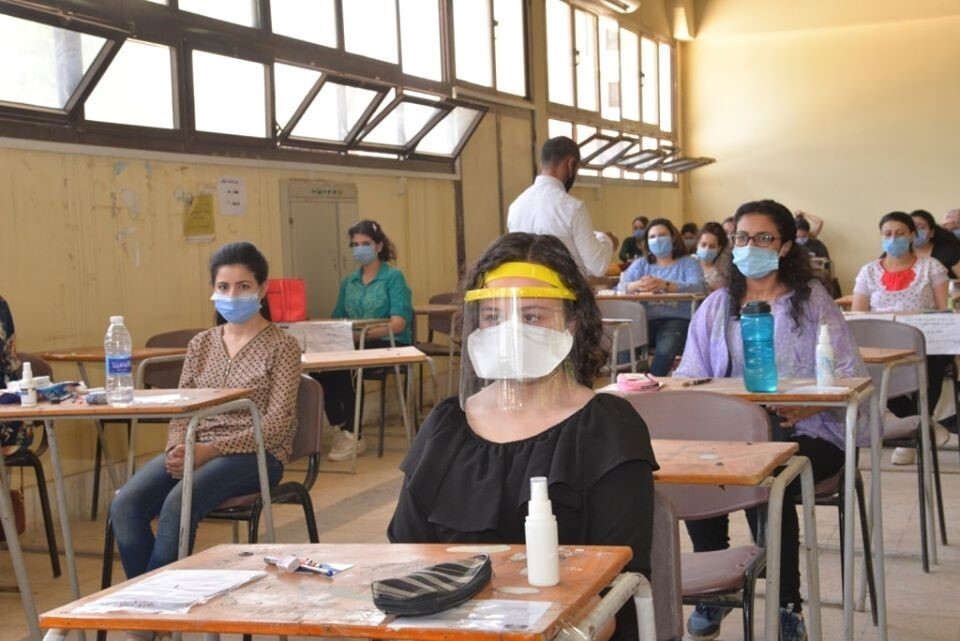Photo credit: Tarek Abd El-Galil

## EGYPT

The Egyptian government has approved draft legislation enabling public universities to set the time required for a student to graduate in hours rather than academic years. Officials say this will allow some students to complete their degrees in three years instead of four and so reduce the cost of higher education to the state. To read more, click here.

 table div table+table+table+table+table+table+table+table+table+table+table+table+table+table+table+table+table+table+table+table+table+table+table+table div table{width:100%;padding:0}table div table+table+table+table+table+table+table+table+table+table+table+table+table+table+table+table+table+table+table+table+table+table+table+table div table img{width:96.23%;padding:0;float:none}table div table+table+table+table+table+table+table+table+table+table+table+table+table+table+table+table+table+table+table+table+table+table+table+table div table td{width:100%;padding:0 1.88% 18px}/* styles */# GTEC Deputy Director General Denies Claims of Discrimination by Technical Universities

Deputy Director General of the Ghana Tertiary Education Commission or GTEC, Dr Ahmed Jinapor Abdulai, has urged staff and faculty of technical universities to ignore erroneous claims that they are discriminated against compared to traditional universities. To read more, click here.

 table div table+table+table+table+table+table+table+table+table+table+table+table+table+table+table+table+table+table+table+table+table+table+table+table+table+table div table{width:100%;padding:0}table div table+table+table+table+table+table+table+table+table+table+table+table+table+table+table+table+table+table+table+table+table+table+table+table+table+table div table img{width:96.23%;padding:0;float:none}table div table+table+table+table+table+table+table+table+table+table+table+table+table+table+table+table+table+table+table+table+table+table+table+table+table+table div table td{width:100%;padding:0 1.88% 18px}/* styles */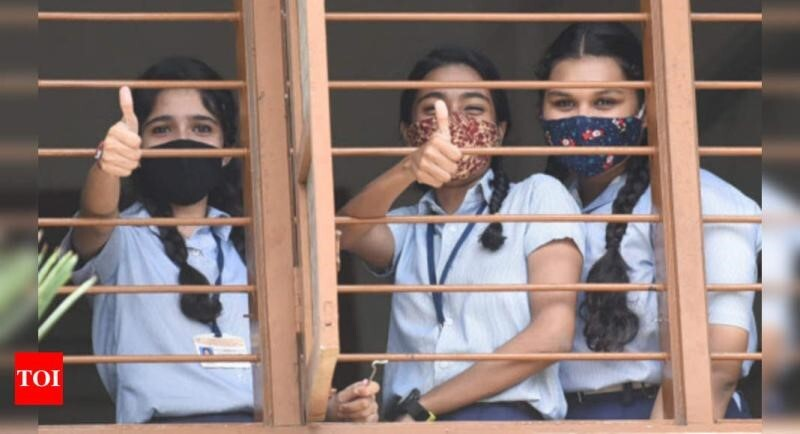Photo credit: Times of India

# CBSE Announces Results for 2021 Class 10

The Central Board of Secondary Education (CBSE) on Tuesday announced the Class 10 result 2021 on its office website. To read more, click here.

 table div table+table+table+table+table+table+table+table+table+table+table+table+table+table+table+table+table+table+table+table+table+table+table+table+table+table+table+table+table div table{width:100%;padding:0}table div table+table+table+table+table+table+table+table+table+table+table+table+table+table+table+table+table+table+table+table+table+table+table+table+table+table+table+table+table div table img{width:96.23%;padding:0;float:none}table div table+table+table+table+table+table+table+table+table+table+table+table+table+table+table+table+table+table+table+table+table+table+table+table+table+table+table+table+table div table td{width:100%;padding:0 1.88% 18px}/* styles */Photo credit: PHOTO | CYRIL NDEGEYA | NATION

# Private Schools Set to Close

Private schools in Rwanda are on the verge of closing down due to low patronage, while schools that have managed to stay open were struggling to meet their operational costs. To read more, click here.

 table div table+table+table+table+table+table+table+table+table+table+table+table+table+table+table+table+table+table+table+table+table+table+table+table+table+table+table+table+table+table+table+table div table{width:100%;padding:0}table div table+table+table+table+table+table+table+table+table+table+table+table+table+table+table+table+table+table+table+table+table+table+table+table+table+table+table+table+table+table+table+table div table img{width:96.23%;padding:0;float:none}table div table+table+table+table+table+table+table+table+table+table+table+table+table+table+table+table+table+table+table+table+table+table+table+table+table+table+table+table+table+table+table+table div table td{width:100%;padding:0 1.88% 18px}/* styles */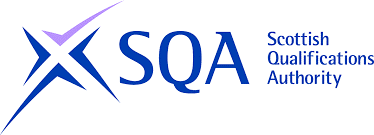# SQA to be replaced as part of sweeping reforms

The Scottish Qualifications Authority (SQA) is to be replaced as part of wide-sweeping reforms in the wake of an international report into the school education system. To read more, click here.

 table div table+table+table+table+table+table+table+table+table+table+table+table+table+table+table+table+table+table+table+table+table+table+table+table+table+table+table+table+table+table+table+table+table+table div table{width:100%;padding:0}table div table+table+table+table+table+table+table+table+table+table+table+table+table+table+table+table+table+table+table+table+table+table+table+table+table+table+table+table+table+table+table+table+table+table div table img{width:96.23%;padding:0;float:none}table div table+table+table+table+table+table+table+table+table+table+table+table+table+table+table+table+table+table+table+table+table+table+table+table+table+table+table+table+table+table+table+table+table+table div table td{width:100%;padding:0 1.88% 18px}/* styles */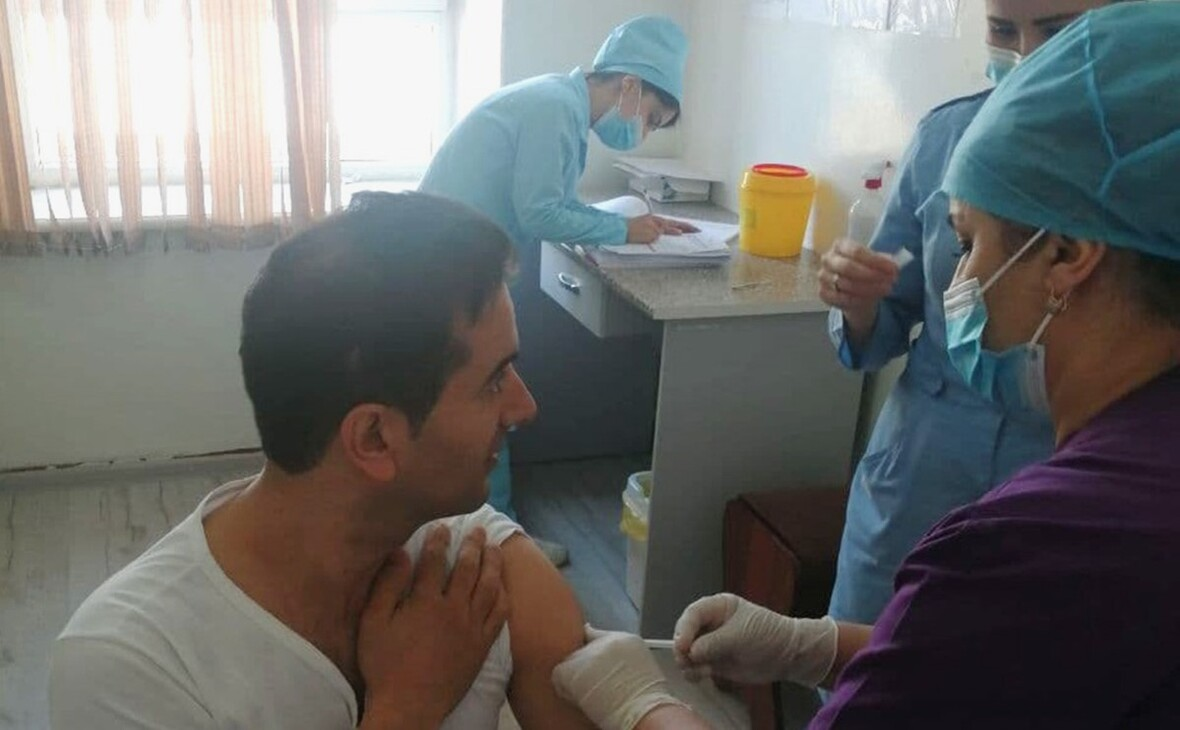Photo credit: adbmch.tj

# Unvaccinated Students Not Allowed to Sit for Entrance Exams

 table div table+table+table+table+table+table+table+table+table+table+table+table+table+table+table+table+table+table+table+table+table+table+table+table+table+table+table+table+table+table+table+table+table+table+table+table+table div table{width:100%;padding:0}table div table+table+table+table+table+table+table+table+table+table+table+table+table+table+table+table+table+table+table+table+table+table+table+table+table+table+table+table+table+table+table+table+table+table+table+table+table div table img{width:96.23%;padding:0;float:none}table div table+table+table+table+table+table+table+table+table+table+table+table+table+table+table+table+table+table+table+table+table+table+table+table+table+table+table+table+table+table+table+table+table+table+table+table+table div table td{width:100%;padding:0 1.88% 18px}/* styles */# Dutch Universities Less Eager for International Students

Universities in the Netherlands have changed their tune when it comes to eagerly attracting international students. Nearly all universities have stopped actively recruiting students from abroad and are now focusing on just providing information. To read more, click here.

 table div table+table+table+table+table+table+table+table+table+table+table+table+table+table+table+table+table+table+table+table+table+table+table+table+table+table+table+table+table+table+table+table+table+table+table+table+table+table+table div table{width:100%;padding:0}table div table+table+table+table+table+table+table+table+table+table+table+table+table+table+table+table+table+table+table+table+table+table+table+table+table+table+table+table+table+table+table+table+table+table+table+table+table+table+table div table img{width:96.23%;padding:0;float:none}table div table+table+table+table+table+table+table+table+table+table+table+table+table+table+table+table+table+table+table+table+table+table+table+table+table+table+table+table+table+table+table+table+table+table+table+table+table+table+table div table td{width:100%;padding:0 1.88% 18px}/* styles */Photo credit: Blank COVID-19 vaccine cards are seen in a photo released by the La Verne Police Department on June 8, 2021

# Fake COVID-19 Vaccine Cards Online Worry College 0fficials

As the delta variant of the coronavirus sweeps across the United States, a growing number of colleges and universities are requiring proof of COVID-19 vaccination for student to attend in-person classes. But the new mandate has opened the door for those opposed to getting the vaccine to cheat the system, according to interviews with students, education and law enforcement officials. To read more, click here.

 table div table+table+table+table+table+table+table+table+table+table+table+table+table+table+table+table+table+table+table+table+table+table+table+table+table+table+table+table+table+table+table+table+table+table+table+table+table+table+table+table+table+table div table{width:100%;padding:0}table div table+table+table+table+table+table+table+table+table+table+table+table+table+table+table+table+table+table+table+table+table+table+table+table+table+table+table+table+table+table+table+table+table+table+table+table+table+table+table+table+table+table div table img{width:96.23%;padding:0;float:none}table div table+table+table+table+table+table+table+table+table+table+table+table+table+table+table+table+table+table+table+table+table+table+table+table+table+table+table+table+table+table+table+table+table+table+table+table+table+table+table+table+table+table div table td{width:100%;padding:0 1.88% 18px}/* styles */# FILMS & DOCUMENTARIES

## For your viewing pleasure, we’ve selected the following documentaries, available through our partner Video Project: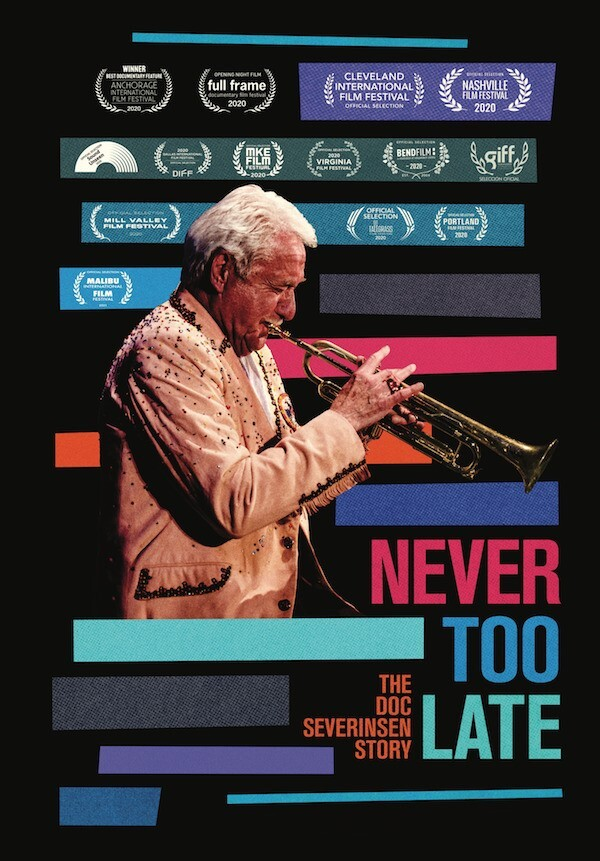/* styles */ Never too late: The Doc Severinsen Story - After three decades as the colorful bandleader to The Tonight Show Starring Johnny Carson, master trumpeter Doc Severinsen defies nature with a relentless schedule of touring and performing into his nineties. A musical icon traces his groundbreaking career and personal trials underscoring a life of inspiration and obsession. To view, click here. "As a document of a major figure in the history of American television and intersections between that medium and music, its value is clear. Visually, the documentary adeptly weaves together recent footage with older archival film, video, and still images. Of course, students of the trumpet may find this documentary attractive, but its strongest academic appeal likely applies to those exploring American television history and its key performers." — Educational Media Reviews Online Click here, to view.
 table div table+table+table+table+table+table+table+table+table+table+table+table+table+table+table+table+table+table+table+table+table+table+table+table+table+table+table+table+table+table+table+table+table+table+table+table+table+table+table+table+table+table+table+table+table+table div table{width:100%;padding:0}table div table+table+table+table+table+table+table+table+table+table+table+table+table+table+table+table+table+table+table+table+table+table+table+table+table+table+table+table+table+table+table+table+table+table+table+table+table+table+table+table+table+table+table+table+table+table div table img{width:96.23%;padding:0;float:none}table div table+table+table+table+table+table+table+table+table+table+table+table+table+table+table+table+table+table+table+table+table+table+table+table+table+table+table+table+table+table+table+table+table+table+table+table+table+table+table+table+table+table+table+table+table+table div table td{width:100%;padding:0 1.88% 18px}/* styles */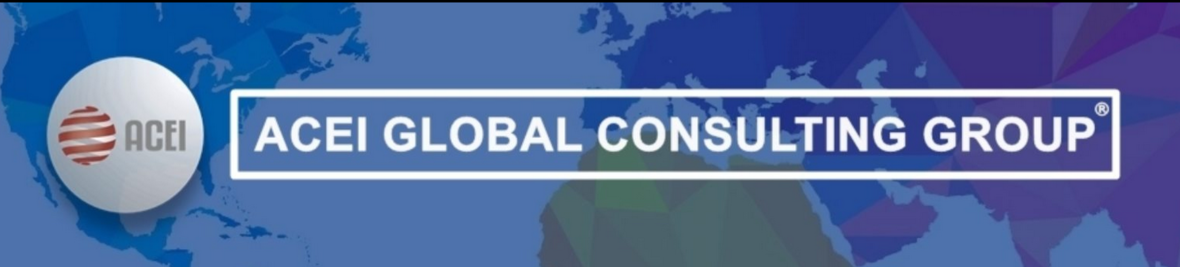table div table+table+table+table+table+table+table+table+table+table+table+table+table+table+table+table+table+table+table+table+table+table+table+table+table+table+table+table+table+table+table+table+table+table+table+table+table+table+table+table+table+table+table+table+table+table+table+table+table+table div table{width:100%;padding:0}table div table+table+table+table+table+table+table+table+table+table+table+table+table+table+table+table+table+table+table+table+table+table+table+table+table+table+table+table+table+table+table+table+table+table+table+table+table+table+table+table+table+table+table+table+table+table+table+table+table+table div table img{width:96.23%;padding:0;float:none}table div table+table+table+table+table+table+table+table+table+table+table+table+table+table+table+table+table+table+table+table+table+table+table+table+table+table+table+table+table+table+table+table+table+table+table+table+table+table+table+table+table+table+table+table+table+table+table+table+table+table div table td{width:100%;padding:0 1.88% 18px}/* styles */table div table+table+table+table+table+table+table+table+table+table+table+table+table+table+table+table+table+table+table+table+table+table+table+table+table+table+table+table+table+table+table+table+table+table+table+table+table+table+table+table+table+table+table+table+table+table+table+table+table+table+table+table div table{width:100%;padding:0}table div table+table+table+table+table+table+table+table+table+table+table+table+table+table+table+table+table+table+table+table+table+table+table+table+table+table+table+table+table+table+table+table+table+table+table+table+table+table+table+table+table+table+table+table+table+table+table+table+table+table+table+table div table img{width:96.23%;padding:0;float:none}table div table+table+table+table+table+table+table+table+table+table+table+table+table+table+table+table+table+table+table+table+table+table+table+table+table+table+table+table+table+table+table+table+table+table+table+table+table+table+table+table+table+table+table+table+table+table+table+table+table+table+table+table div table td{width:100%;padding:0 1.88% 18px}/* styles */